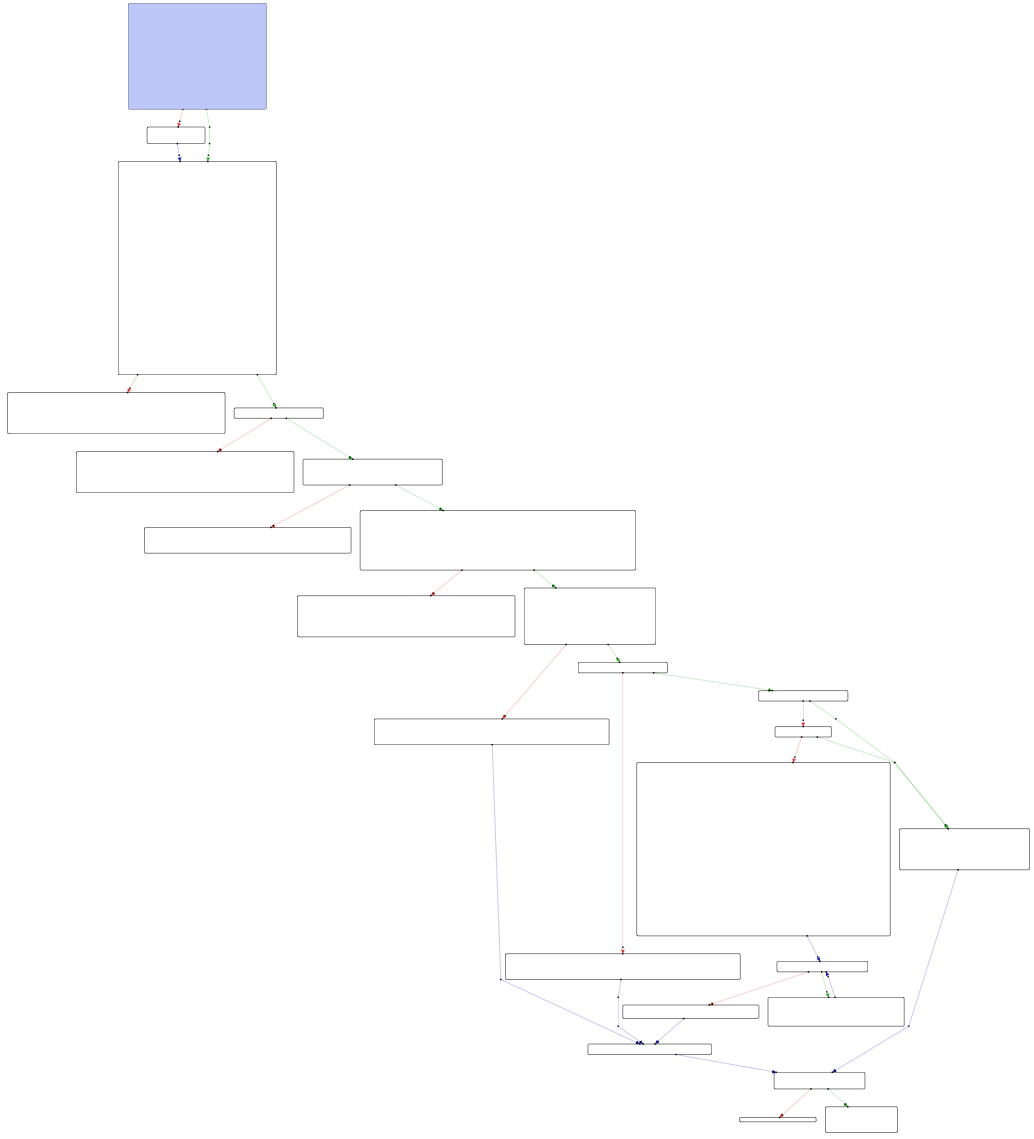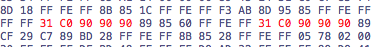# Crackme 11: ELF, Random

## Mar 19, 2018

Link: https://www.root-me.org/en/Challenges/Cracking/ELF-Random-Crackme (binary) This crackme is significantly more complex than the previous ones:A quick look shows a protection against debuggers using `ptrace(0,0,1,0) < 0`. Let’s start by breaking it so that we can make progress:

``````loc_8048ad3:
mov        dword [esp+0x10124+var_10118], 0x0 ; CODE XREF=main+517
mov        dword [esp+0x10124+var_1011C], 0x1
mov        dword [esp+0x10124+var_10120], 0x0
mov        dword [esp+0x10124+var_10124], 0x0 ; argument "__request" for method j_ptrace
call       j_ptrace     ; ptrace
test       eax, eax
jns        loc_8048b2c
``````

We change the `JNS` (0x79) into a `JMP` (0xEB) and save the binary. We can now debug it:

``````Reading symbols from ./Crackme.dbg...done.
gdb\$ r
Starting program: ./Crackme.dbg

** Bienvenue dans ce challenge de cracking **

[-] Try again

[Inferior 1 (process 27) exited with code 0377]
--------------------------------------------------------------------------[regs]
``````

With this new binary, let’s take a look at the initial setup in `loc_80488f3`. There’s first a `memset` of 0xffff bytes to zero, then a call to `time(0)` (the current time), the result of which is passed to `srand`. We then call `getpid` and store it in `ebp` - 0x100a0:

``````lea        edx, dword [ebp-0x1007b] ; CODE XREF=main+79
mov        eax, 0xffff
mov        dword [esp+0x10120+var_10118], eax ; argument "__n" for method j_memset
mov        dword [esp+0x10120+var_1011C], 0x0 ; argument "__c" for method j_memset
mov        dword [esp+0x10120+var_10120], edx ; argument "__s" for method j_memset
call       j_memset     ; memset
mov        dword [ebp-0x1009c], 0x0
mov        dword [esp+0x10120+var_10120], 0x0 ; argument "__timer" for method j_time
call       j_time       ; time
mov        dword [esp+0x10120+var_10120], eax ; argument "__seed" for method j_srand
call       j_srand      ; srand
call       j_getpid     ; getpid
mov        dword [ebp-0x100a0], eax
call       j_rand       ; rand
``````

The return value of `rand` is used in a series of numeric calculations, some involving floating-point arithmetic. It’s pretty complex so let’s not get into it in details. This is followed by a call to `sprintf` that builds a string with the pattern “%lld%s”, with a reference to “_VQLGE_TQPTYD_KJTIV_”. Let’s run through it a couple of times, and see what comes out of `sprintf`:

``````\$ ltrace ./Crackme.dbg 2>&1 | grep sprintf
sprintf("5884452_VQLGE_TQPTYD_KJTIV_", "%lld%s", 5884452, nil) = 27
\$ ltrace ./Crackme.dbg 2>&1 | grep sprintf
sprintf("2008856_VQLGE_TQPTYD_KJTIV_", "%lld%s", 2008856, nil) = 27
\$ ltrace ./Crackme.dbg 2>&1 | grep sprintf
sprintf("653161_VQLGE_TQPTYD_KJTIV_", "%lld%s", 653161, nil) = 26
``````

As these examples show, the random value is eventually transformed into an integer that gets prepended to “_VQLGE_TQPTYD_KJTIV_”. This is followed by a call to `malloc(0xffff)`, and then `malloc(0x15)`. We `strcmp` the formatted string with our input and store the result in `ebp`-0x7c before storing their respective lengths in two other local variables:

``````lea        eax, dword [ebp-0x1007b] ; CODE XREF=sub_8048b20+100
mov        dword [esp+0x10124+var_10120], eax ; argument "__s2" for method j_strcmp
lea        eax, dword [ebp-0x7c]
mov        dword [esp+0x10124+var_10124], eax ; argument "__s1" for method j_strcmp
call       j_strcmp     ; strcmp
mov        dword [ebp-0x10080], eax
lea        eax, dword [ebp-0x7c]
mov        dword [esp+0x10124+var_10124], eax ; argument "__s" for method j_strlen
call       j_strlen     ; strlen
mov        dword [ebp-0x10088], eax
lea        eax, dword [ebp-0x1007b]
mov        dword [esp+0x10124+var_10124], eax ; argument "__s" for method j_strlen
call       j_strlen     ; strlen
mov        dword [ebp-0x10084], eax
``````

If the two lengths are different, we jump to an error message:

``````mov        eax, dword [ebp-0x10088]
cmp        eax, dword [ebp-0x10084]
``````

If the result of `strcmp` was less than or equal to zero, we also jump to a failure message (see reference to `\$ebp`-0x10080 above):

``````loc_8048c50:
cmp        dword [ebp-0x10080], 0x0 ; CODE XREF=sub_8048b20+250
``````

Following these branches, we notice that the `strcmp` needs to have returned zero, and that the lengths need to match as well (this check is redundant):

``````mov        eax, dword [ebp-0x10088]
cmp        eax, dword [ebp-0x10084]
jne        loc_8048ded
``````

To crack tis program, we’ll need to predict the value of this random string, or make it predictable. We can patch the binary again, removing the call to `rand()` and replacing its value with zero. Here is the call instruction and the offset of the next one:

``````0804893b         call       j_rand                                              ; rand
08048940         mov        ecx, eax
``````

We have 5 bytes between the two. We can insert `xor eax, eax` which is [0x31, 0xc0] and add 3 NOPs [0x90, 0x90, 0x90]. We now have, with addresses:

``````0804893b         xor        eax, eax
0804893d         nop
0804893e         nop
0804893f         nop
08048940         mov        ecx, eax
``````

We need to do the same to `getpid` and return zero there too. Instead of:

``````08048930         call       j_getpid                                            ; getpid
08048935         mov        dword [ebp-0x100a0], eax
``````

We’ll edit 08048930 and replace 5 bytes there too. Here’s the hex view:Let’s save the binary again and try it a few times under `ltrace`:

``````\$ ltrace ./Crackme.norand 2>&1 | grep sprintf
sprintf("0_VQLGE_TQPTYD_KJTIV_", "%lld%s", 0, nil) = 21
\$/# ltrace ./Crackme.norand 2>&1 | grep sprintf
sprintf("0_VQLGE_TQPTYD_KJTIV_", "%lld%s", 0, nil) = 21
\$ ltrace ./Crackme.norand 2>&1 | grep sprintf
sprintf("0_VQLGE_TQPTYD_KJTIV_", "%lld%s", 0, nil) = 21
``````

Our string is now predictable, and we can provide the same value as input:

``````\$ ./Crackme.norand

** Bienvenue dans ce challenge de cracking **